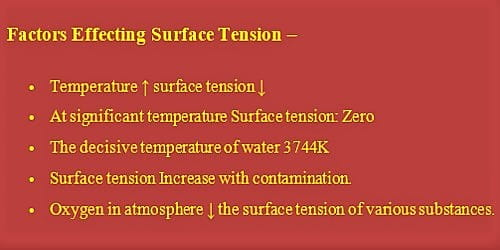# Factors that Influencing the Surface Tension of a Liquid

The force with which the surface molecules are held simultaneously is called surface tension. Theoretical Definition: “If a straight line is considered on the surface of a liquid, then on both sides of that line the tangential force per unit length that act on the surface of the liquid is called surface tension”.

Factors influencing the surface tension of a liquid:

The surface tension of a liquid depends on the following factors:

• Contamination

If the liquid surface gets contaminated by oily or greasy substances its surface tension in general decreases. For example, a vastly soluble essence like sodium chloride (HCl) when dissolved in water increases the surface tension of water. But the cautiously soluble substances like phenol when dissolved in water decreases the surface tension of water.

• Presence of dissolved substances

If a substance is dissolved in a liquid the surface tension of that liquid is changed. Adding chemicals to a liquid will alter its surface tension characteristics. If inorganic substances are dissolved in liquid surface tension of the liquid increases but if organic substances are dissolved the surface tension decreases. The consequence of adding a dissimilar chemical to a substance, and thereby changing its surface tension, is demonstrated by the example of putting soap (a surfactant) in water to decrease the surface tension, which allows the dirt on your hands to more simply mix with the water.

• Temperature

The surface tension of a liquid is largely dependent on temperature. Generally, the surface tension decreases with the increase of temperature and increases with the decrease of temperature. In surface tension a critical temperature disappears i.e. is absent or zero. Exceptions are noticed only in case of melted copper and cadmium. Within a small range of temperature, the relation between surface tension Tt and temperature t; is –

Tt = T0 (1 – at)

here, Tt = Surface tension at temperature t0C, T0 =  Surface tension at 00C and α is the temperature coefficient of surface tension.

As temperature decreases, surface tension increases. On the other hand, as surface tension decreases strongly; as molecules become more active with an increase in temperature becoming zero at its boiling point and evaporation at significant temperature. The surface tension of a liquid decreases with increase in temperature. The surface tension of a liquid becomes zero at its boiling point and vanishes at a decisive temperature.

• Medium above the liquid

The surface tension of a liquid depends on the nature of the medium above the free surface. For example- surface tension of water is 70 x10-3 Nm-1, when vapor is above the water surface. On the other hand, it is 72 x 10-1 Nm-1, when air is in contact with water.

Most inorganic salts somewhat elevate surface tension of water even though potassium permanganate lowers it. In liquid-liquid and solid-liquid systems, dissolved substances usually lower interfacial tension.

• Oxidation

Oxidation straight affects surface tension. As surface tension increases, intermolecular forces increase. Oxygen in the atmosphere is known to decrease the surface tension of various substances.

• Electrification

When a liquid is electrified, surface tension decreases. Since external force acts on the liquid surface due to electrification, so an area of the liquid surface increases which acts against contraction phenomenon of surface tension. Hence, it also decreases.

• The Presence of Impurities

The presence of impurities on the surface of, or dissolved in, a substance straight affects the surface tension of the liquid. The surface tension of water, for example, will increase when extremely soluble impurities are added to it. Impurities present in a liquid significantly influence surface tension. A highly soluble material like salt increases the surface tension whereas sparingly soluble substances like soap decrease the surface tension.

Key points of Factors affecting surface tension:

• Temperature ↑ surface tension ↓
• At significant temperature Surface tension: Zero
• The decisive temperature of water 3744K
• Surface tension Increase with contamination.